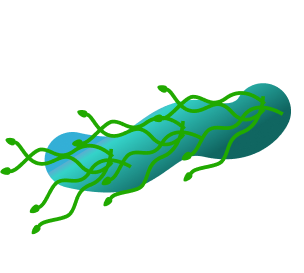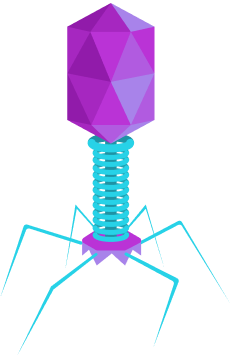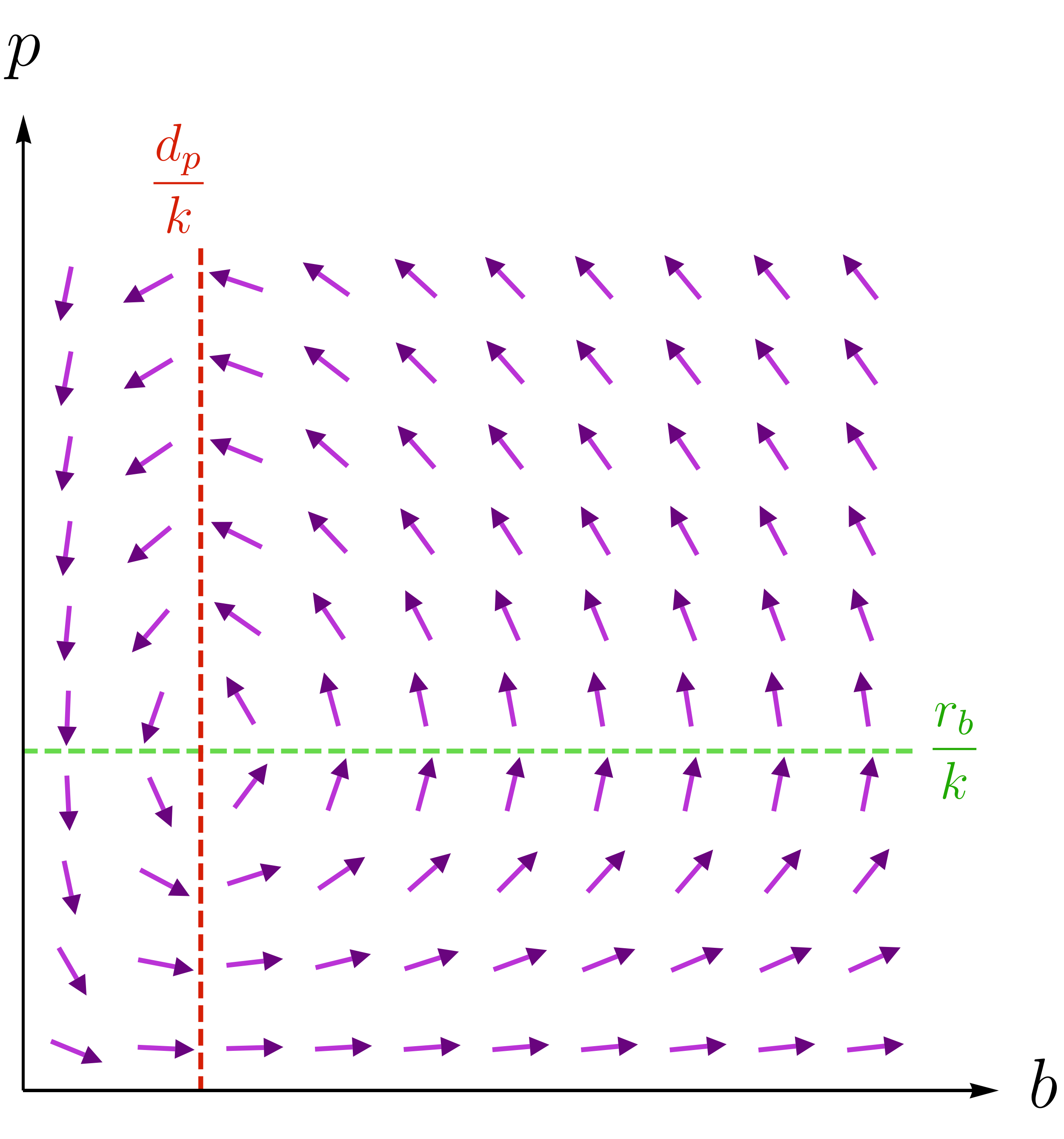### Differential Equations II

Our course focuses on just two types of problems.

The first type involves nonlinear systems of differential equations, or nonlinear equations for short; we'll talk about the second type later.

Nonlinear equations model things like competitive behavior, lasers, and even the weather!

This quiz gives a lightning tour of just some of the topics we'll cover.

Let's get started by answering a basic question: “What are nonlinear equations?”

# Nonlinear Equations in a Nutshell

To understand nonlinear equations, let's remember what linear equations are all about.

A differential equation is linear if any two solutions $y_{1}$ and $y_{2}$ combine like $y_{1} + c y_{2} ,$ $c\in \mathbb{R}$ to make another. Nonlinear equations are the ones that don't have this property.

Let's look at an example. Bacteria are single-cell critters that reproduce by splitting.A cartoon picture of a bacterium

Put a few in a very large petri dish with a great deal of food, and their population $b(t)$ grows like $b'(t) = r_{b} b(t), r_{b} > 0:$ more bacteria means more splitting, meaning more bacteria...

Is the equation $b'(t) = r_{b} b(t)$ linear or nonlinear?

# Nonlinear Equations in a Nutshell

Now for our next question: “Why do we need nonlinear equations?”

The function $b(t) = b(0) e^{r_{b} t}$ grows incredibly fast and without end, so the model predicts that the rampaging bacteria will overrun the cosmos given enough time!This isn't very realistic, but the model ignores the limited amount of food, so we shouldn't take it too seriously in the long term. As we'll see later, there's a simple fix that caps the population: $b'(t) = r_{b} b(t)\big[ \textcolor{#D61F06}{M-b(t)}\big]$ is called the logistic equation, and the constant $M$ is the carrying capacity.

Is the logistic equation linear or nonlinear?

# Nonlinear Equations in a Nutshell

So nonlinear equations are needed to make realistic models. The logistic equation is an improvement over the original bacteria equation $b' = r_{b} b,$ but it's also harder to solve.

The logistic equation $b' = r_{b} b [ M - b ]$ is actually separable, so with some effort we find $b(t) = \frac{ M ~ b(0) ~ e^{r_{b} M t} }{M + b(0)\big[ e^{r_{b} M t} - 1\big] }.$ The interactive plot below shows this solution (red) together with the original $b(t) = b(0) e^{ r_{b} t}.$

Notice that $b(t) = M$ is a special solution: it doesn't change with time, and it attracts others in the long term $\big($i.e. $\lim\limits_{t \to \infty} b(t) = M$ always$\big).$

Constant solutions are generally called equilibria; like the logistic equation's carrying capacity $M$ here, they often tell us what matters most, the long-term behavior of solutions.

# Nonlinear Equations in a NutshellNot only are equilibria important in the long term, but they're also the easiest solutions to find! To show what we mean, let's introduce a phage, which is a virus that kills a bacterium to reproduce.

The animation shows two scenarios for a phage infection: in one, the initial population of the bacteria is high, and in the other, it's low.

The more bacteria/phages there are, the faster the phage spreads, so the product $k\,b(t) p(t)$ does a pretty good job quantifying the spread rate; $k>0$ measures the phage's effectiveness.

If the phages increase at the same rate as they cull the bacteria, we have a Lotka-Volterra pair $\begin{cases} b'(t) = \underbrace{r_{b} b(t)}_{\text{normal growth rate}} - \underbrace{k\, p(t) b(t)}_{\text{culling part}} \\\\ p'(t) = \underbrace{k\, p(t) b(t)}_{\text{phage reproduction rate}} \end{cases}$ if we ignore phage death. Select all options that are equilibria for this nonlinear system.

# Nonlinear Equations in a Nutshell

Select one or more

Equilibria are typically the best we can hope for if our aim is to write down closed-form solutions to a nonlinear system. Equilibria luckily contain a wealth of info!

For instance, equilibria help us plot direction fields, like this one for our bacteria and phages:

Later, we'll use equilibria to make direction fields from scratch; for now, it's enough to see that a direction field is a very handy visual tool since solution curves (red) must “follow its arrows.”

Experiment with different initial values $\big(b(0), p(0)\big)$ (green dot), and use the direction field to make a guess about the long-term survival of the bacteria.

# Nonlinear Equations in a Nutshell

Solutions of nonlinear equations aren't always driven to an equilibrium, though.

Let's say our phage pals aren't immortal but die off at the rate $- d_{p}p(t).$ We have a new Lotka-Volterra pair $\begin{cases} b'(t) = \underbrace{r_{b} b(t)}_{\text{normal growth rate}} - \underbrace{k\, p(t) b(t)}_{\text{culling part}} \\\\ p'(t) = \underbrace{-d_{p} p(t)}_{\text{phage death rate}} + \underbrace{k\, p(t) b(t)}_{\text{phage reproduction rate}}, \end{cases}$ which has an equilibrium at $b = \frac{d_p}{k}, \, p = \frac{r_{b}}{k}.$ Its direction field is plotted below.Lotka-Volterra direction field. The equilibrium sits where the dashed lines meet.

How would you describe the behavior of its solutions?

# Nonlinear Equations in a Nutshell

Cycles are solutions that close on themselves, like the ones in the last phage-bacterium model.

Sometimes, a nonlinear pair can have an isolated cycle that attracts or repels solutions like an equilibrium point. We call it a limit cycle because of how it affects other solutions as $t \to \infty.$

The pursuit problem is a classic example of a limit cycle. Later on, we'll meet a hapless duck named Happy, who's pursued relentlessly around the edge of a circular pond by Terry, who can't swim nearly as fast.If Terry gives chase when Happy has a significant head start, then the nonlinear equations guiding their motion quickly bring them to a never-ending cycle of pursuit.

# Nonlinear Equations in a Nutshell

The variety of long-term behavior becomes far richer when we include more variables and nonlinear equations. The Lorenz system is probably the most famous example of this: \begin{aligned} x'(t) &= \sigma (y- x) \\ y'(t) &= x \left( \rho - z\right) - y \\ z'(t) &= x y - b z. \end{aligned} ,\ \ \ \rho, \sigma, b > 0 These equations were originally designed to be a simple model of convection rolls in fluids.Water warmed by the stove top rises and is replaced by descending colder water; the result is a convection roll. Lorenz's model relates $x,$ the rate of convection, to $y$ and $z,$ the horizontal and vertical temperature variations.

We'll show later that when the constants $\rho, \sigma,$ and $b$ are chosen just right, all solutions are attracted towards a very strange-looking set that's neither an equilibrium nor a cycle. The animation shows the progress of two such solutions.

The points trace paths $\big(x(t),y(t),z(t)\big)$ through time $t$ given by the Lorenz equations, and start with nearly identical initial conditions. How would you describe their long-term behavior?

# Nonlinear Equations in a Nutshell

In a nutshell, when a set of differential equations has two solutions that can't be combined linearly to make a third, it's nonlinear.

Many realistic models in science and engineering are made up of nonlinear equations, and it's often the case that we're really only interested in such a model's long-term behavior.

Solutions may be attracted to or repelled by equilibria, limit cycles, or more exotic strange sets as we saw with the Lorenz equations.

There's so much to explore in the world of nonlinear equations, and we've barely just begun.

In the next quiz, we introduce partial differential equations, the other focus of our course.

# Nonlinear Equations in a Nutshell

×